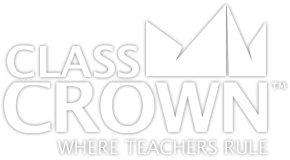# Math Worksheets

## Riddle-Me-Worksheets

### Math worksheets with riddles to keep kids motivated.

Key Features
•Self checking
•Problem Solving motivation
•Common core aligned
•High quality design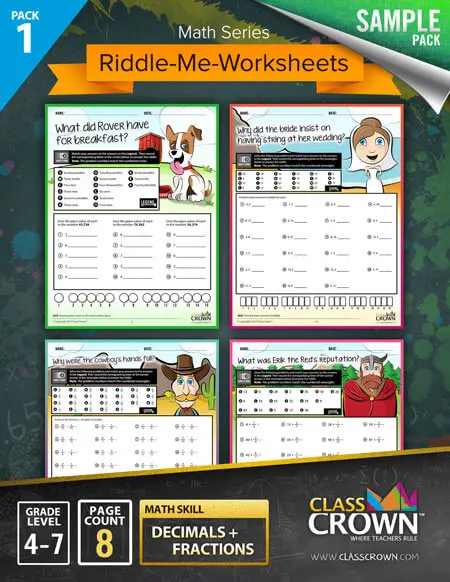##### Sample Pack 1
###### Decimals & Fractions Worksheets
• P1  – Naming place value to the thousandths place
• P2 – Adding and subtracting decimals
• P3 – Finding the least common multiple
• P4 – Converting mixed numbers to improper fractions
• P5 – Subtracting fractions with like denominators
• P6 – Adding and subtracting fractions word problems
• P7 – Multiplying whole numbers by fractions
• P8 – Multiplying whole numbers by fractions, word problems
Included Math Worksheets: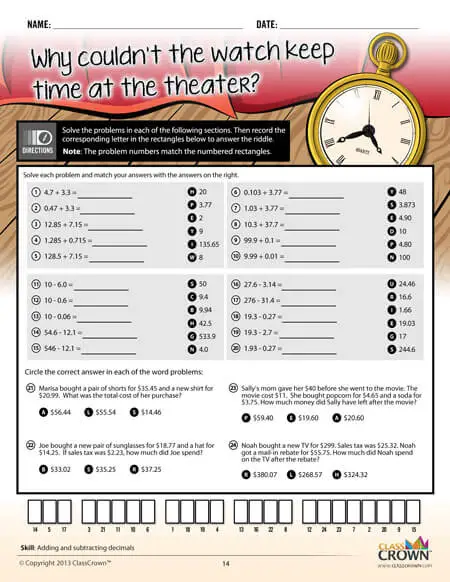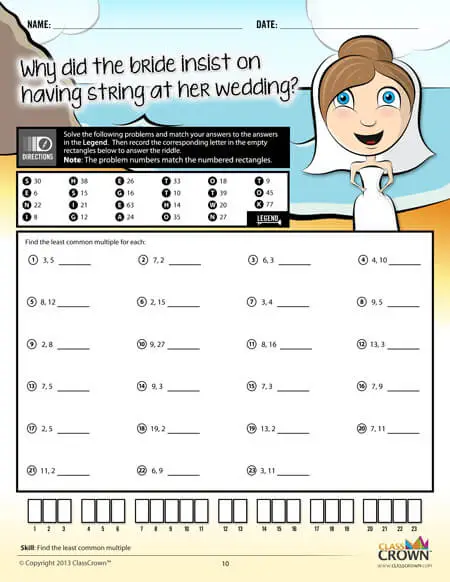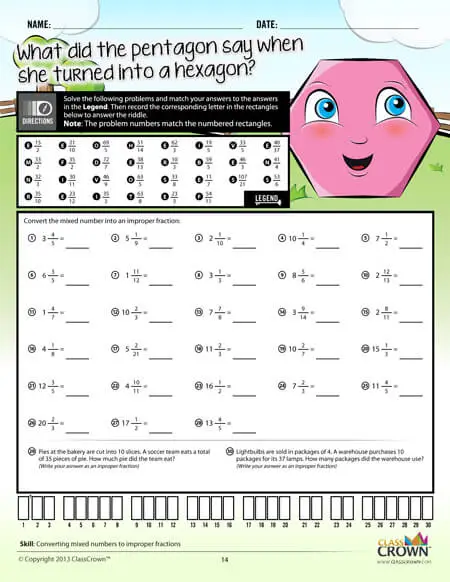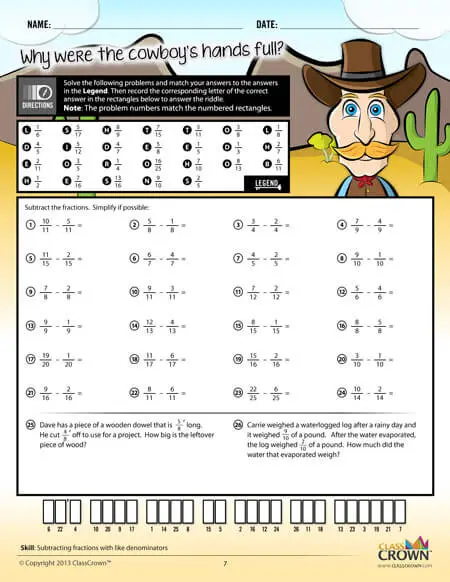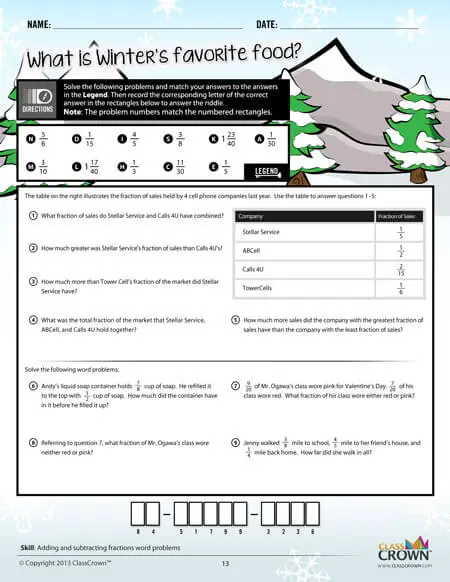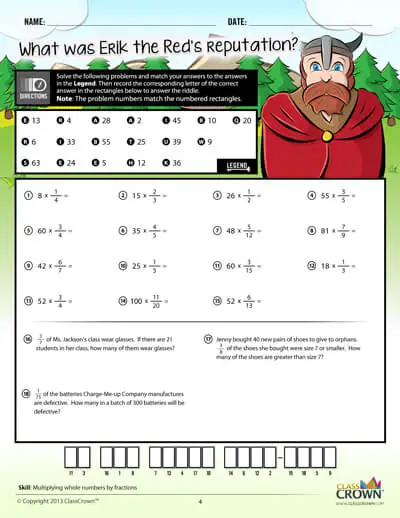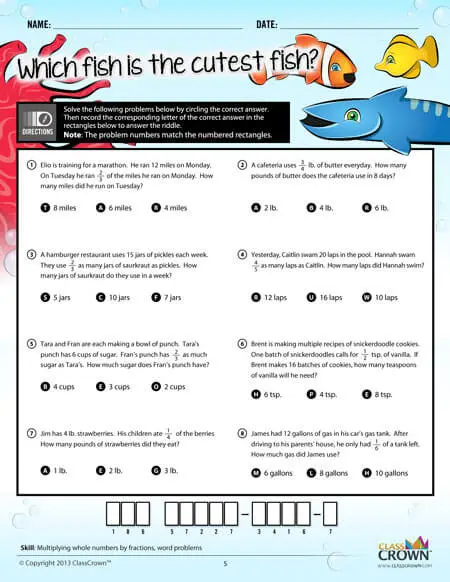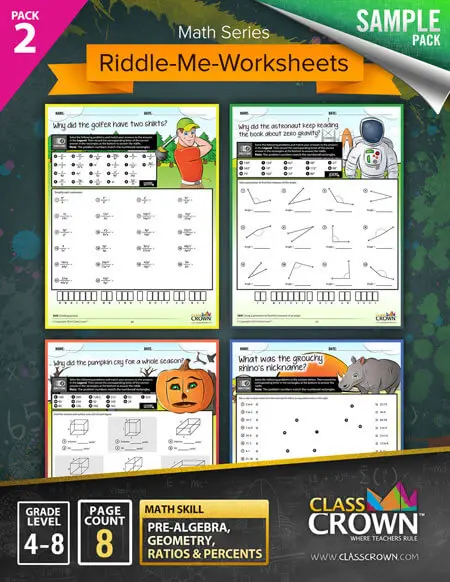##### Sample Pack 2
###### Pre-algebra, Geometry, Ratios & Percents
• P1  – Dividing powers
• P2 – Subtracting integers
• P3 – Using a protractor to find the measure of an angle
• P4 – Finding unknown angles in triangles and quadrilaterals
• P5 – Finding the volume & surface area of rectangular prisms
• P6 – Finding surface area of cylinders, rectangular prisms, and right square pyramids
• P7 – Equivalent ratios
• P8 – Proportions
Included Math Worksheets: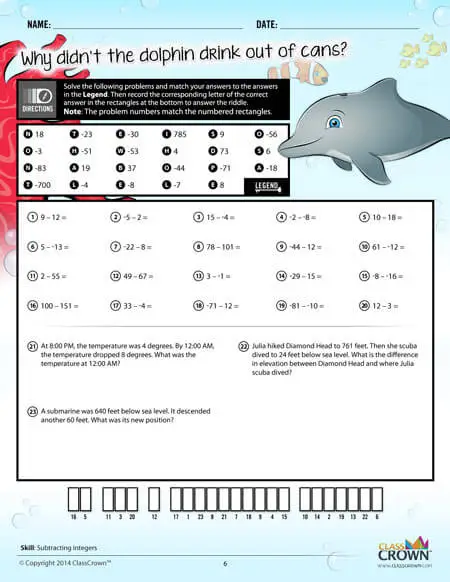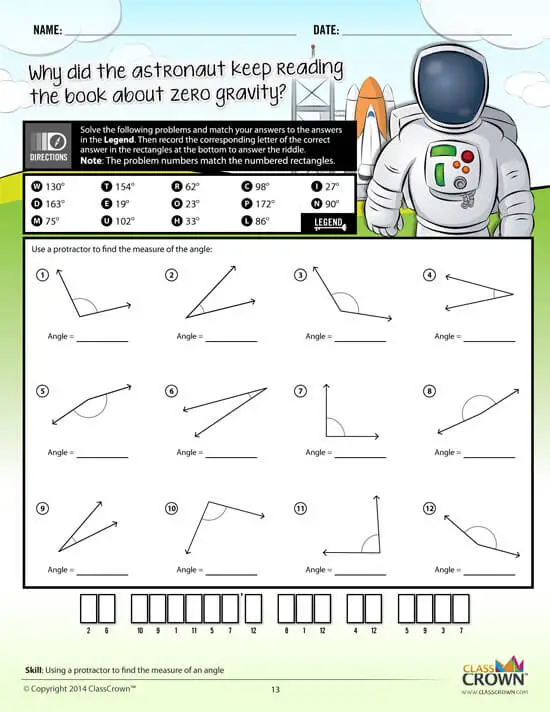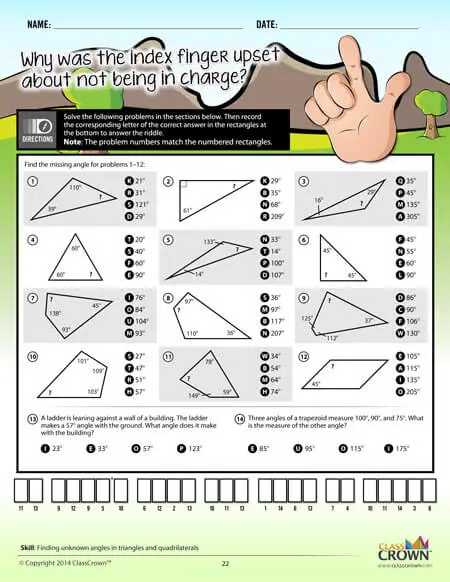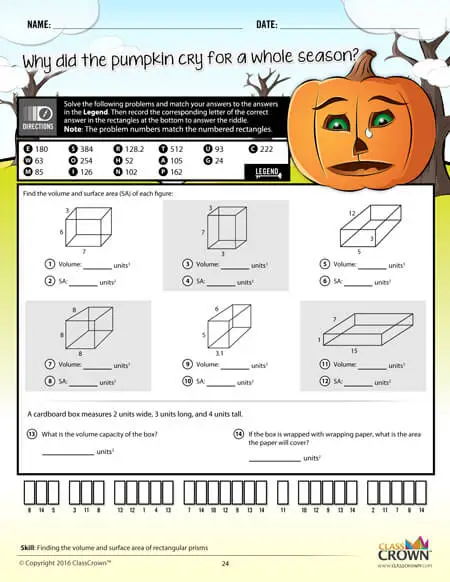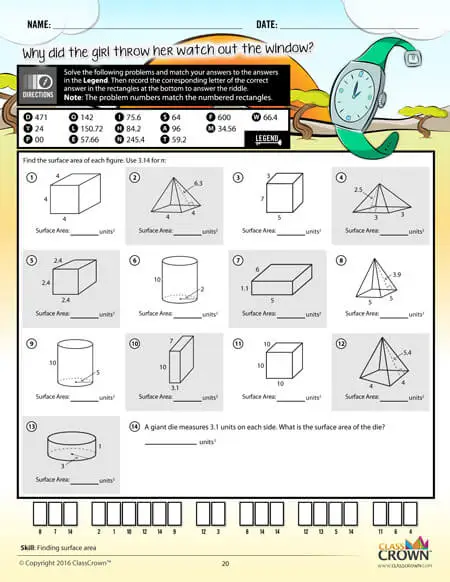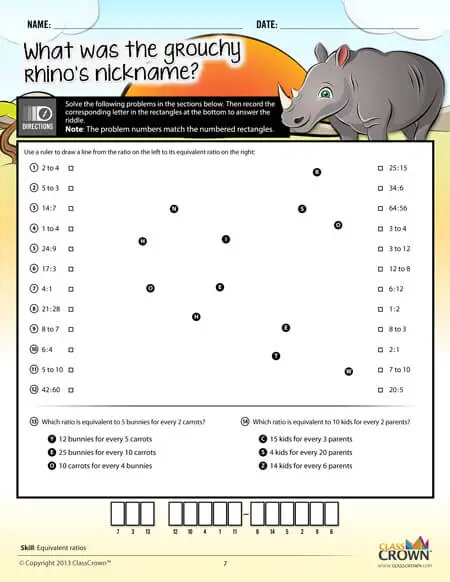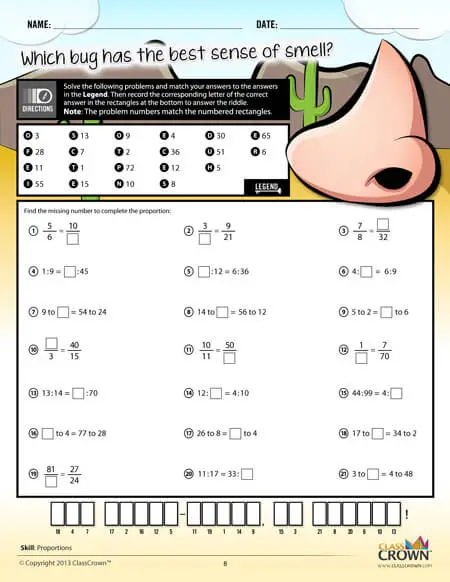Self Checking

Our math worksheets introduce a puzzle aspect to math, giving students immediate feedback as to whether or not they are solving problems correctly. If the answer to the riddle isn't spelled correctly, the student knows which problems he's made an error on.Fun Puzzle AspectImmediate Feedback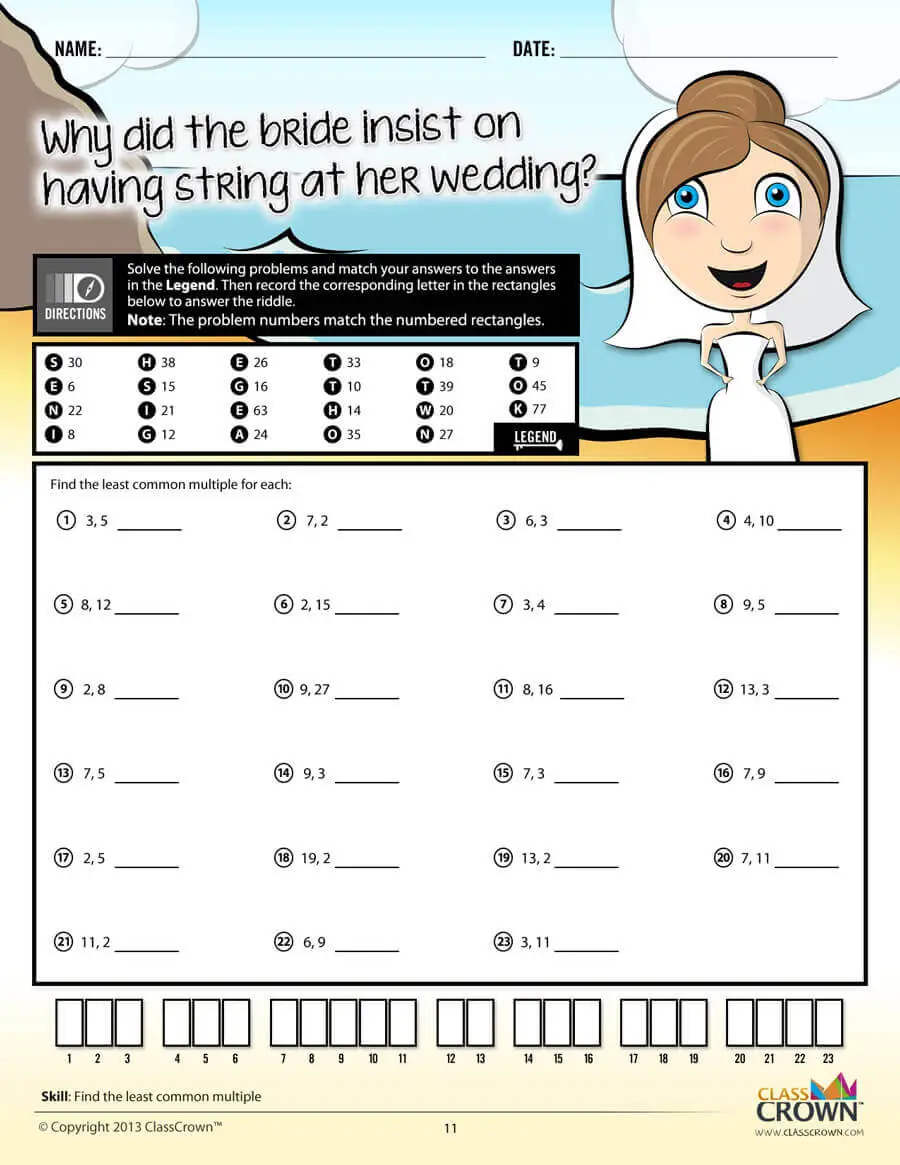Problem Solving Motivation

Each math worksheet contains a riddle that the student solves by completeing all the problems on the worksheet. This keeps kids motivated to complete each problem so that they can find the answer to the riddle.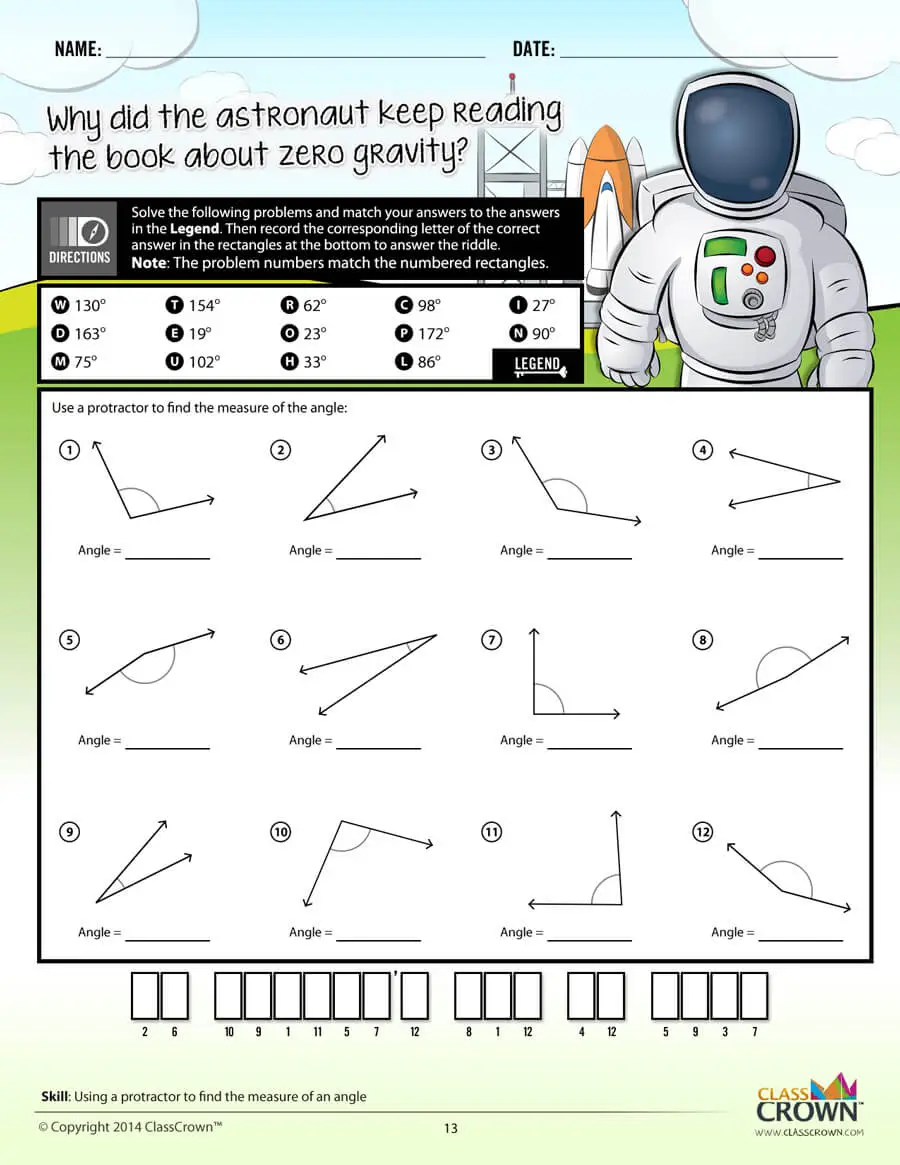Common Core Aligned

All our math worksheet packs are designed with Common Core in mind. That way you don’t have to worry about whether your math ciriculum is aligned or not when you incorpoate ClassCrown Riddle-Me-Worksheets in your lesson plans.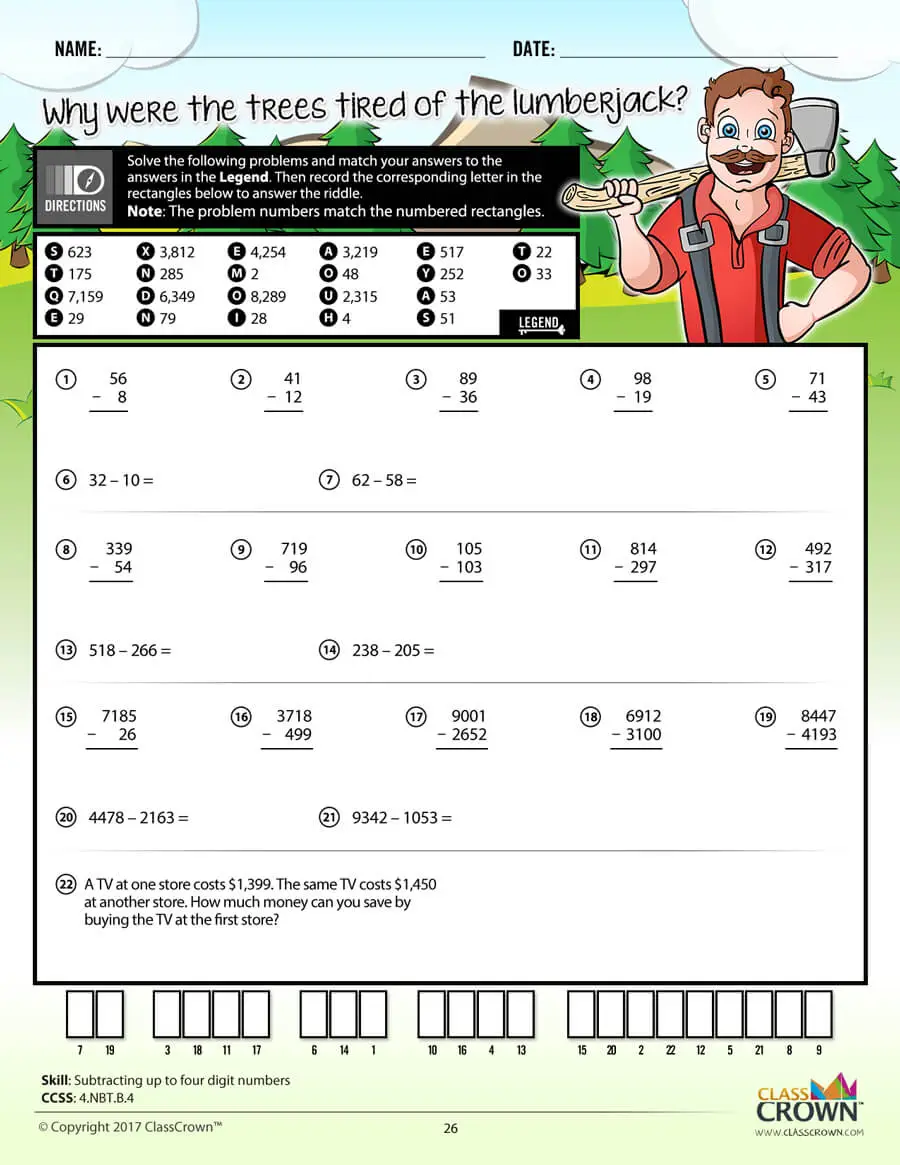High Quality Design

Each page of our math worksheets has been produced in high resolution at 144 dpi and designed in full, vibrant color for maximum quality. They look stunning whether you are printing in color or black and white.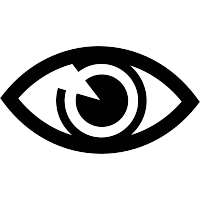High Resolution
(144 dpi)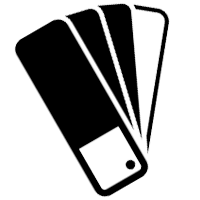Stunning Color & Clarity